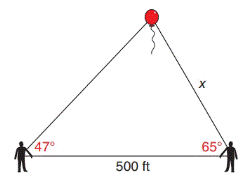Chapter 11.4, Problem 30EElementary Geometry For College St...

7th Edition
Alexander + 2 others
ISBN: 9781337614085

Solutions

Chapter
SectionElementary Geometry For College St...

7th Edition
Alexander + 2 others
ISBN: 9781337614085
Textbook Problem

In Exercises 29 to 34, use the Law of Sines or the Law of Cosines to solve each problem. Angle measures should be found to the nearest degree and areas and distances to the nearest tenth of a unit.Phil and Matt observe a balloon. They are 500  ft apart, and their angles of observation are 47 ∘ and 65 ∘ , as shown. Find the distance x from Matt to the balloon.To determine

To find:

The distance x from matt to the balloon.

Explanation

Formula:

The Law of sines,

sinαa=sinβb=sinγc

Where α, β, and γ is the angle of the triangle, and a, b, and c is the sides of the triangle.

Calculation:

Given,

Phil and Matt observe a balloon.

They are 500 ft apart, and their angles of observation are 47 and 65 are shown below.

Let A and B were Phil and Matt’s position respectively and C is the balloon’s position with angle α.

Then, AB¯=500 ft , BC¯=x , B=65 , and A=47

a=500 ft , b=x , β=65 , and γ=47

Find x:

For any triangle,

α+β+γ=180

Here,  β=65  and  γ=47

α+47+65=180

α+112=180

Still sussing out bartleby?

Check out a sample textbook solution.

See a sample solution

The Solution to Your Study Problems

Bartleby provides explanations to thousands of textbook problems written by our experts, many with advanced degrees!

Get Started

Fill in each blank: 54in.=ft

Elementary Technical Mathematics

Prove that limx21x=12.

Single Variable Calculus

Solve the inequality. 50. |x 6| 0.1

Single Variable Calculus: Early Transcendentals, Volume I